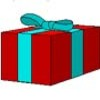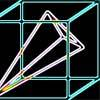#### You may also likeA box of size a cm by b cm by c cm is to be wrapped with a square piece of wrapping paper. Without cutting the paper what is the smallest square this can be?### Plane to See

P is the midpoint of an edge of a cube and Q divides another edge in the ratio 1 to 4. Find the ratio of the volumes of the two pieces of the cube cut by a plane through PQ and a vertex.### Four Points on a Cube

What is the surface area of the tetrahedron with one vertex at O the vertex of a unit cube and the other vertices at the centres of the faces of the cube not containing O?

# Cubestick

##### Age 16 to 18Challenge Level

Glue three cubes together to make a cuboid of dimensions $3$ by $1$ by $1$. Label $4$ points on the cuboid with coordinates $A = (0,0,0)$, $B=(1,1,1)$, $C=(2,1,0)$ and $D=(3,1,0)$. Now the challenge is to find at least two different ways to calculate the angles $\angle ABC$ and $\angle ABD$.